Next: Compound Pendula Up: Simple Harmonic Oscillation Previous: LC Circuits

# Simple Pendula

Consider a compact mass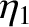suspended from a light inextensible string of length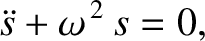, such that the mass is free to swing from side to side in a vertical plane, as shown in Figure 5. This setup is known as a simple pendulum. Let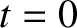be the angle subtended between the string and the downward vertical. The stable equilibrium state of the system corresponds to the situation in which the mass is stationary, and hangs vertically down ( i.e.,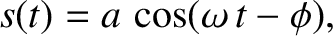). The angular equation of motion of the pendulum is (Fowles and Cassiday 2005)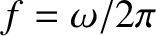(48)

where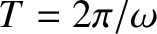is the moment of inertia of the mass, and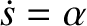the torque acting about the suspension point. For the case in hand, given that the mass is essentially a point particle, and is situated a distancefrom the axis of rotation (i.e., from the suspension point), it follows that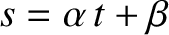(ibid.).

The two forces acting on the mass are the downward gravitational force,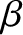, where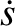is the acceleration due to gravity, and the tension,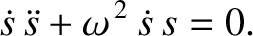, in the string. However, the tension makes no contribution to the torque, because its line of action passes through the suspension point. From elementary trigonometry, the line of action of the gravitational force passes a perpendicular distance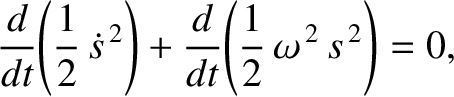from the suspension point. Hence, the magnitude of the gravitational torque is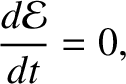. Moreover, the gravitational torque is a restoring torque: that is, if the mass is displaced slightly from its equilibrium position (i.e.,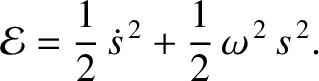) then the gravitational torque acts to push the mass back towards that position. Thus, we can write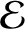(49)

Combining the previous two equations, we obtain the following angular equation of motion of the pendulum,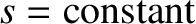(50)

Unlike all of the other time evolution equations that we have examined, so far, in this chapter, the preceding equation is nonlinear [because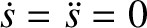], which means that it is generally very difficult to solve.

Suppose, however, that the system does not stray very far from its equilibrium position (). If this is the case then we can expand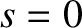in a Taylor series about. (See Appendix B.) We obtain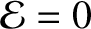(51)

If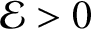is sufficiently small then the series is dominated by its first term, and we can write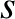. This is known as the small angle approximation. Making use of this approximation, the equation of motion (50) simplifies to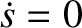(52)

where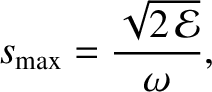(53)

Equation (52) is the simple harmonic oscillator equation. Hence, we can immediately write its solution in the form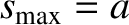(54)

where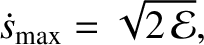and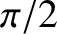are constants. We conclude that the pendulum swings back and forth at a fixed angular frequency,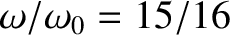, that depends onand, but is independent of the amplitude,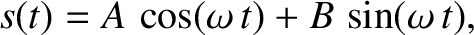, of the motion. This result only holds as long as the small angle approximation remains valid. It turns out thatis a good approximation provided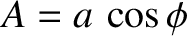. Hence, the period of a simple pendulum is only amplitude independent when the (angular) amplitude of its motion is less than about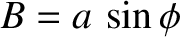.Next: Compound Pendula Up: Simple Harmonic Oscillation Previous: LC Circuits
Richard Fitzpatrick 2013-04-08